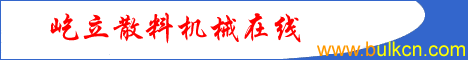How to calculate supporting force by geometry                                                       Li Yimin 2001-1-20                            return   return home For the foundation design and the check of traveling gantry's rigidity and strength, the wheel loads usually are calculated in the design of Gantry crane and bucket wheel stacker  reclaimer. Three-points or Four-points usually support gantries of above-mentioned machines. The gravity centers of such machines change according to luffing angle and slewing angle. By the method of geometry can figure out each supporting force comparative easily.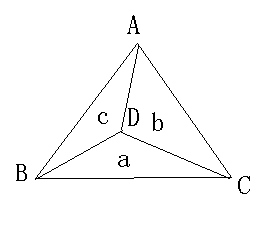fig 1.Three-points style 1.The supporting force calculation of Three-points style Three-points style is three legs support gantry. As showed in figure 1. Three-points support A, B and C showed in figure 1 are the positions of three-supporting points. D is the gravity center. The triangle composed by A, B, C is a random triangle, D is a random point in the random triangle. Link point D with points A,B,C respectively to get lines AD,BD,CD, to divided the big triangle into three smaller triangles, the area of triangle ACD is Sb; the area of triangle ABD is Sc; the area of triangle BCD is Sa; the area of triangle ABC is S. areas of above mentioned triangles can measure out directly by drawing soft CAD. Assume: the load on gravity center D is G: Supporting forces on legs are NA, NB, NC We get: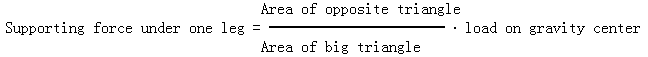(1) Area of triangle on the opposite of any point in formula (1) is the area of smaller triangle on the opposite of any angle point of bigger triangle. For example, triangle BCD is the opposite triangle of point A.   By formula (1)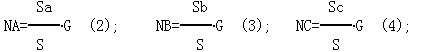I.e., supporting force of any point in Three supporting points is direct proportional to the area of opposite triangle and load, the opposite triangle is triangle on the opposite of this point, which get by divided the bigger triangle from the gravity center.      2.The calculation of four-supporting point's style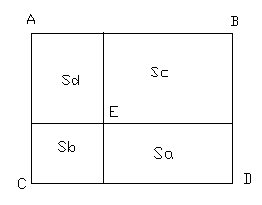Figure 2  four points support four-points is four legs support gantry, as showed in figure 2, A, B, C, D are four supporting points. The shape surrounded by point A, B, C, D is rectangle or square, E is the gravity center in the rectangle. Draw perpendicular lines to four side lines of the rectangle, assume the are composed by A, B, C, D is S, as showed in figure 2, areas of four smaller rectangles are Sa,Sb,Sc,Sd respectively. CAD could measure out the areas of above-mentioned rectangle directly. Assume: the load on gravity center E is G;   Supporting forces from legs are NA，NB，NC，ND：  Get: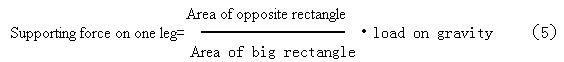The area of small opposite rectangle in formula (5) is the area of a rectangle which on the opposite of a angle point of the big rectangle.  For example, the area of small rectangle on the side of point A is Sa.  By formula (5), we getI.e., the supporting force of some point of four supporting points is directly proportional to the area of the opposite small rectangle and the load, is on the inverse proportion with the area surrounded by four supporting points. Calculate supporting force by the geometry method is simple and direct, specially by CAD, no wonder three supporting points or four supporting points, make a assistant line after determine the gravity center, can tell the supporting force easily by compare the areas.                                                                                              By Li yinmin  Jan.20th,2001 Copyright  ©  by BULK-ONWEB, BULK MACHINERY TECHNIQUE  2001-2005         版权所有：《散料机械技术》《屹立散料机械在线》          未经许可授权不可转载和使用本站稿件内容